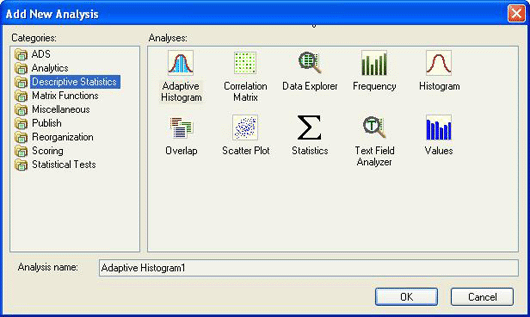Descriptive Statistics - Teradata Warehouse Miner - 5.4.4

Teradata Warehouse Miner User Guide - Volume 1Introduction and Profiling

prodname
vrm_release
5.4.4
category
User Guide
featnum
B035-2300-077K
The descriptive statistics available with Teradata Warehouse Miner provide a variety of functions to statistically analyze and explore a Teradata database. Descriptive statistical analysis is valuable for the following reasons.
• It can provide business insight in its own right.
• It uncovers data quality issues which, if not corrected or compensated for, can jeopardize the accuracy of any analytic models that are based on the data.
• It isolates the data that should be used in building analytic models. For example, outlying values should sometimes be excluded from a model; in other cases, these values might be required to solve a particular business problem. Further, some statistical processes used in analytic modeling require a certain type of distribution of data.
Descriptive statistical analysis can determine the suitability of various data elements for model input and can suggest transformations that may be required for these data elements.

In the case of the Descriptive Statistics, NULL values are handled through the generated SQL’s aggregate functions. In this case, SQL ignores the NULL value and adjusts the number of observations in its calculation. This effectively provides a listwise deletion of NULL values.

The following are the descriptive statistical functions currently available in Teradata Warehouse Miner:
• Adaptive Histogram — Determine the distribution of a numeric column(s) giving counts, sub-binning column(s) with higher counts and determining data spikes.
• Correlation Matrix — Build and view a correlation matrix.
• Data Explorer — Automated exploration of any number of tables or views within an entire database.
• Frequency — Compute frequency of column values or multi-column combined values. Optionally, compute frequency of values for pairs of columns in a single column list or two column lists, and generate simple statistics for any other column within a table.
• Histogram — Determine the distribution of a numeric column(s) giving counts with optional overlay counts and statistics.
• Overlap — Count overlapping column values in combinations of tables (i.e., find “key” values in common between tables).
• Scatter Plot — Plot sampled values of two to three variables in 2-D or 3-D.
• Statistical Analysis — Determine any of the following descriptive statistics for numeric column(s):
1. Minimum Value
2. Maximum Value
3. Mean Value
4. Standard Deviation
5. Skewness
6. Kurtosis
7. Standard Mean Error
8. Coefficient of Variance
9. Variance
10. Sum
11. Uncorrected Sums of squares
12. Corrected Sums of squares
13. Values Count
14. Modal Value
15. Percentiles
16. Top 5/Bottom 5 Rank and Values
• Text Field Analyzer — Analyze character data and help distinguish whether the field is a numeric type, a date, a time, a timestamp, or character data.
• Values Analysis — Count the number of values of various kinds for a given column or columns, including:
1. Number of Rows
2. Rows with Non-NULL Values
3. Rows with NULL Values
4. Unique Values
5. Rows with Value ‘0’
6. Rows with a Positive Value
7. Rows with a Negative Value
8. Rows Containing Blank Values

In order to add a Descriptive Statistical analysis to a Teradata Warehouse Miner Data Mining Project, create a new analysis with any of the mechanisms described in Using Teradata Warehouse Miner. This will produce the following dialog.

Add New Analysis dialogDouble-click or highlight the desired analysis, optionally change the default name and click OK. Each of these specific analyses are described in detail in the subsequent sections.